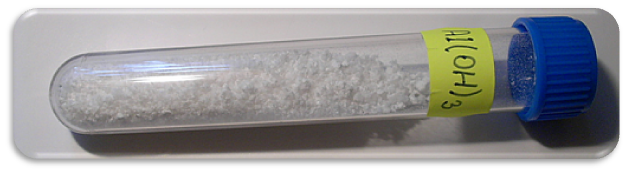## The Solubility of Amphoteric Metal Hydroxides

#### Learning Objective

• Recall the solubility properties of amphoteric metal hydroxides.

#### Key Points

• Hydroxides of the metals of Group 3 and higher tend to be weakly basic and most display an amphoteric nature, meaning that they can neutralize acids and bases.
• Amphoteric metal hydroxides can form a series of oxyanions (anions containing oxygen), depending on the number of protons removed by hydroxide ions.
• Most amphoteric compounds are also amphiprotic, meaning that they can act as either a proton donor or a proton acceptor.

#### Terms

• amphiproticBeing able to both donate and accept a proton, and thus being able to react both as an acid and a base; amphoteric.
• amphotericHaving the characteristics of both an acid and a base, and capable of reacting as either; amphiprotic.

## Amphoteric Metal Hydroxides

The oxides and hydroxides of the metals in Group 3 and higher tend to be weakly basic and mostly display an amphoteric nature. Most of these compounds are so slightly soluble in water that their acidic or basic character is only obvious in their reactions with strong acids or bases. In general, these compounds tend to be more basic than acidic; thus, the oxides and hydroxides of aluminum, iron, and zinc all dissolve in mildly acidic solution:

$Al(OH)_3 +3 H^+\rightarrow Al^{3+}(aq) +3H_2O$ ;

$Al(OH)_3 (s) + OH^- \rightarrow Al(OH)_4^- (aq)$Aluminum hydroxide solidAluminum hydroxide is an amphoteric metal hydroxide.

$Zn(OH)_2 +3 H^+ \rightarrow Zn^{2+}(aq) +2H_2O$ ;

$Zn(OH)_2 (s) +2 OH^- \rightarrow Zn(OH)_3^{4- } (aq)$

The product ions in the second set of reactions (after the semi-colon) are complex ions, known as aluminate and zincate.

## Amphoteric Versus Amphiprotic

An amphoteric substance is one that can act as either an acid or a base. An amphiprotic substance can act as either a proton donor or a proton acceptor. Since acids are proton donors while bases are proton acceptors, it therefore follows that all amphiprotic compounds are also amphoteric. An example of an amphoteric compound that is not amphiprotic is ZnO, which can act as an acid even though it has no protons to donate.

An example of an amphoteric and amphiprotic substance is beryllium hydroxide (Be(OH)2):

$Be(OH)_2 + 2HCl \rightarrow BeCl_2 + 2H_2O$

As a base, it accepts a proton from HCl, forming beryllium chloride (BeCl2) and water.

$Be(OH)_2 + 2NaOH \rightarrow Na_2[Be(OH)_4], forming \ complex \ ion\ [Be(OH)_4]^{2-}$

In the second example, Be(OH)2 forms a complex ion when excess hydroxide ions are available.